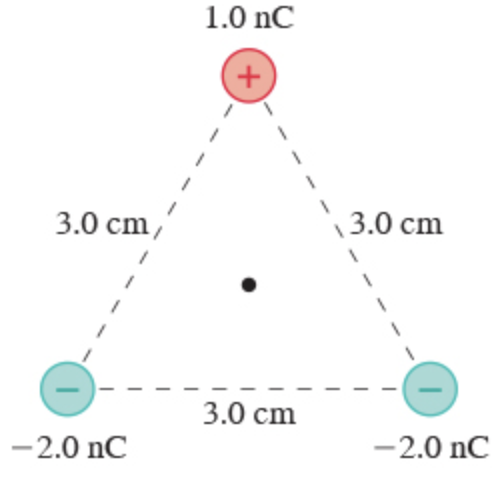In: Physics

# What is the electric potential at the point indicated with the dot in the figure?

What is the electric potential at the point indicated with the dot in the figure?Express your answer to two significant figures and include the appropriate units.

## Solutions

##### Expert Solution

Use the properties of equilateral triangle,

\begin{aligned} r &=\frac{a}{\sqrt{3}} \\ &=\frac{3}{\sqrt{3}} \\ &=\sqrt{3} \mathrm{~cm} \end{aligned}

$$=\sqrt{3} \times 10^{-2} \mathrm{~m}$$

The potential at given point is, $$V=\frac{k q_{1}}{r}+\frac{k q_{2}}{r}+\frac{k q_{3}}{r}$$

$$=\frac{k}{r}\left(q_{1}+q_{2}+q_{3}\right)$$

$$=\frac{9 \times 10^{9}}{\sqrt{3} \times 10^{-2}}\left(1 \times 10^{-9}-2 \times 10^{-9}-2 \times 10^{-9}\right)$$

$$=\frac{9 \times 10^{9} \times 10^{-9} \times(-3)}{\sqrt{3} \times 10^{-2}}$$

$$=\frac{-27}{\sqrt{3}} \times 10^{2}$$

$$=-1558 \mathrm{~V}$$

Thus, the electric potential at the point indicated (rounding off to two significant figures) is $$-1600 \mathrm{~V}$$.